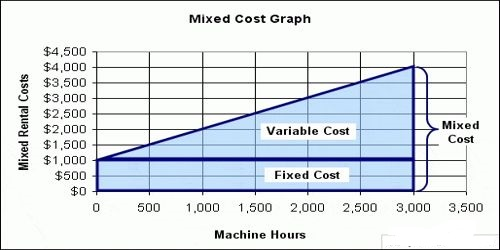Accounting

# Different methods of analyzing Mixed CostDifferent methods of analyzing Mixed Cost

Mixed costs contain elements of both fixed and variable cost behavior. As with step costs, the fixed elements is determined by the planned range of activity level. We know of three methods for separating mixed costs into their fixed and variable cost components:

• Scatter graph by plotting points onto a graph.
• High-low method.
• Regression analysis.

It is wise to prepare the scatter graph even if you use the high-low method or regression analysis. The benefit of the scatter graph is that it allows you to see if some of the plotted points are simply out of line. These points are referred to as outliers and will need to be reviewed and possibly adjusted or eliminated. In other words, you don’t want incorrect data to distort your calculations under any of the three methods.

Let’s assume that a company uses only one type of equipment and it wants to know how much of the monthly electricity bill is a constant amount and how much the electricity bill will increase when its equipment runs for an additional hour. The scatter graph’s vertical or y-axis will indicate the dollars of total monthly electricity cost. Its horizontal or x-axis will indicate the number of equipment hours. For each monthly electricity bill, a point will be entered on the graph at the intersection of the dollar amount of the total electricity bill and the equipment hours occurring between the meter reading dates shown on the electricity bill. If you plot this information for the most recent 12 months, you may see some type of pattern, such as a line that rises as the number of equipment hour’s increase.

If you draw a line through the plotted points and extend the line through the y-axis, the amount where the line crosses the y-axis is the approximate amount of fixed costs for each month. The slope of the line indicates the variable cost per equipment hour. The slope or variable rate is the increase in the total monthly electricity cost divided by the change in the total number of equipment hours.

The high-low calculation is similar but it uses only two of the plotted points: the highest point and the lowest point.

Regression analysis uses all of the monthly electricity bills amounts along with their related number of equipment hours in order to calculate the monthly fixed cost of electricity and the variable rate for each equipment hour. The software can be used for regression analysis and it will also provide statistical insights.

If a scatter graph of data shows no clear pattern, you should not place much confidence in the calculated amount of the fixed cost and variable rate regardless of the method used.